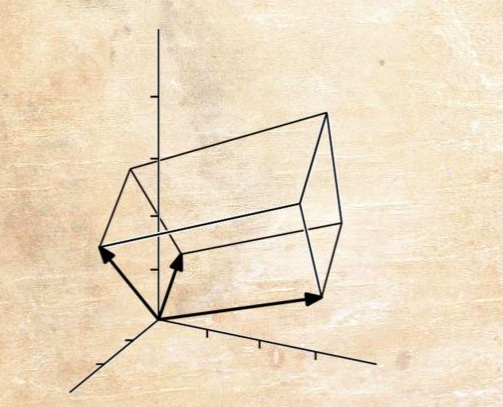# Linear Algebra

These notes have been derived from (i) Essence of Linear Algebra (ii) MIT OCW Linear Algebra, and (iii) Matrix Methods in Machine Learning.### Vector Spaces

This is meant to accompany each corresponding video in the Essence of Linear Algebra Series. Here we study the first eight chapters of the series. These notes are meant as an index (with some additional commentary) for the topics covered in the vidoes and are not meant as a substitute for the videos.

### System of Linear Equations (Part I)

Essence of Linear Algebra talks about the Matrix as a list of column vectors. In this note, we explore the Matrix as a set of linear equations (row-wise). We go over Gaussian Elimination, Matrix Inversion and A = LU Factorization; the topics that were left out of Essence of Linear Algebra videos. They follow Gilbert Strang’s 18.06 Course on MIT OCW. Also note, for now, we only talk about square matrices and systems where num of linear equations is equal to the number of unknowns.

### System of Linear Equations (Part II)

Till now we have only worked with square matrices and linear systems with as many equations as the number of unknowns. Now we step into the realm of non-square matrices. These notes are majorly derived from MIT OCW 18.06 Series.

### Least Squares

We are now equipped with the basics of Linear Algebra. We are familiar with how a matrix can be used to represent a vector space, as well as a system of linear equations. Now let us try to apply this knowledge to learn from data (aka Machine Learning). We will be relying on ECE 532 and MIT 18.06 for the same.

### EigenDecomposition and SVD

In the last note, we looked at our first application of Linear Algebra in Machine Learning. Here we look at SVD, which again has lots of ML applications, one of the most common being PCA. For now, we will conclude with the discussion on SVD, and take up its applications as part of another series.